# BMI Calculation Formula In Ms Excel

You might have come across a task in which you were assigned to make BMI calculations of the supplied numbers, and you may be looking for an efficient approach to accomplish this process rather than doing BMI calculations manually, by which you can also complete that task, but ultimately you will end up exhausted.

But don’t worry; we’ve got your back and brought up a most accessible way to perform BMI calculations in a couple of seconds.

So, without further ado, let’s get started.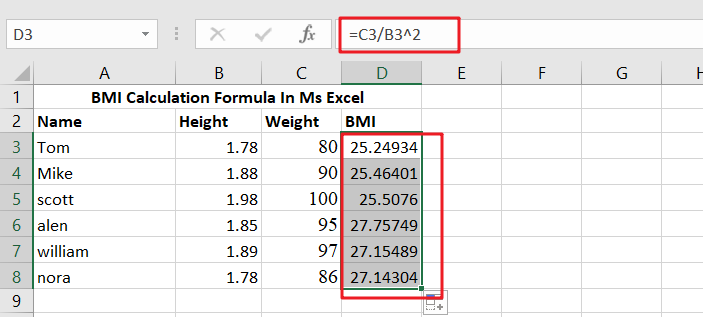## What Exactly Is BMI?

Body Mass Index is an abbreviation for Body Mass Index. It is a simple (and affordable) method of determining body fat based on height and weight. BMI is a screening technique that may determine if a person is underweight, overweight, or obese. BMI, on the other hand, is not a diagnostic tool.

## General Formula

The algorithm below may be used to compute BMI calculations.

`=weight/height^2`

## Explanation of Syntax

You must be aware of the Formula’s syntax to use it to complete your task.

• Division (/): This symbol divides values or integers.

## Summary

This tutorial will look at two different ways to compute BMI (Body Mass Index) in Excel. We first convert height and weight to metric units in the example worksheet before calculating BMI using a conventional metric method. In D3, the Formula is:

`=C3/B3^2`

It delivers an estimated BMI based on a G5 weight and an F5 height## Explanation

It is easier to grasp the application of the above Formula with the assistance of two examples. The first example demonstrates an effective method for calculating BMI (Body Mass Index) in Excel. The typical BMI formula is as follows:

`BMI = weight (kg) / height (m)2`

The second method employed here is to first convert height in inches and feet to meters, and weight in pounds to kilograms, before applying the conventional metric calculation for BMI. This allows for collecting size and weight, generally used units (in the United States), and displaying measured quantities utilized in the computation.

The fundamental difficulty, in this case, is that most Americans still use the US conventional measuring system to record height and weight rather than the metric system. As a result, the initial step is to record this data is regularly used units.

Then, in cell E12, we utilize the CONVERT function twice to compute height in meters:

`=CONVERT(B12,"ft","m")+CONVERT(C12,"in","m")`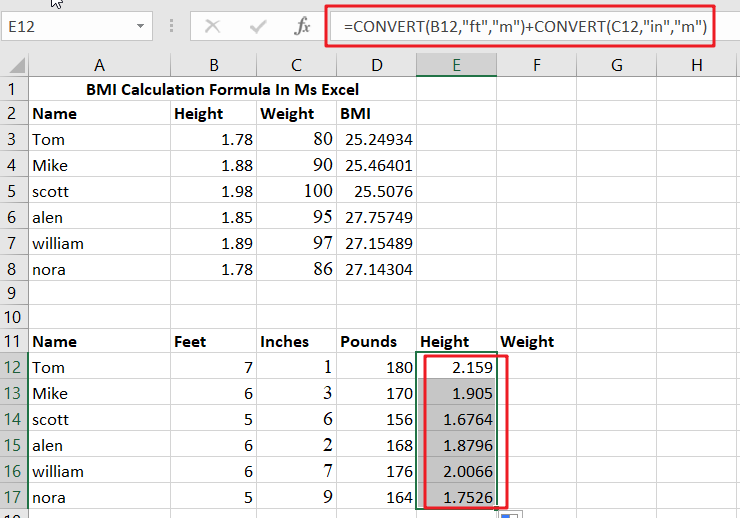The first CONVERT translates feet to meters as follows:

`=CONVERT(B12,"ft","m")/ convert feet to meters`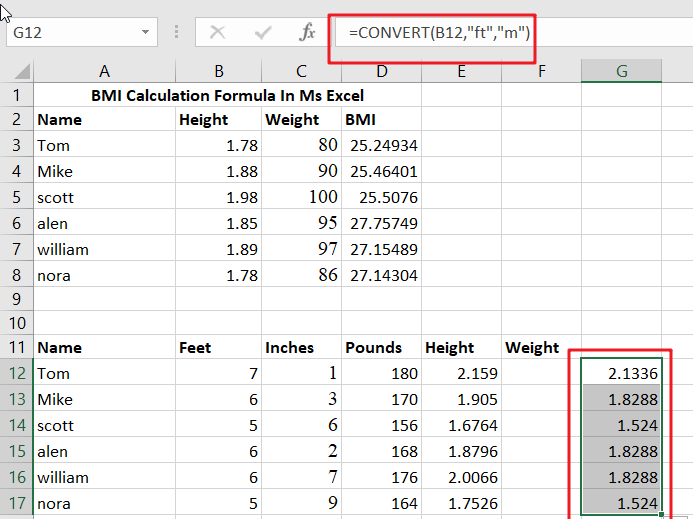The second translates inches to meters as follows:

`=CONVERT(C12,"in","m")/ converting inches to meters`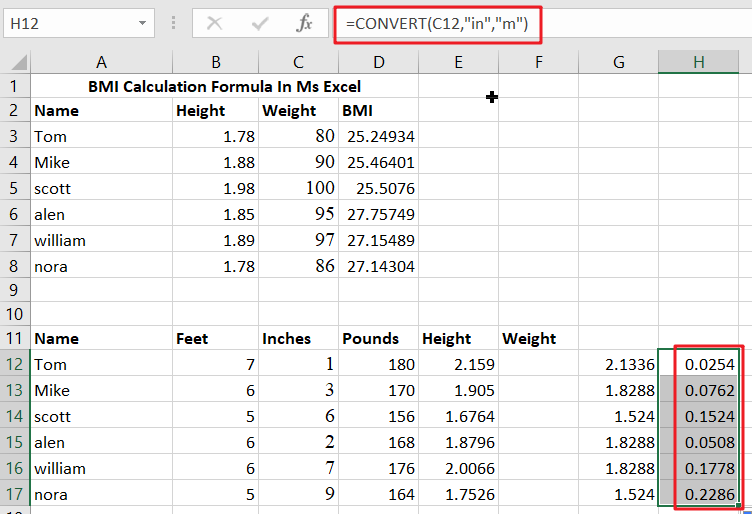The two numbers are then put together to obtain the total height in meters.

In cell F12, we use CONVERT once again to determine the weight in kilograms:

`=CONVERT(D12,"lbm","kg")/ converting pounds to kilograms`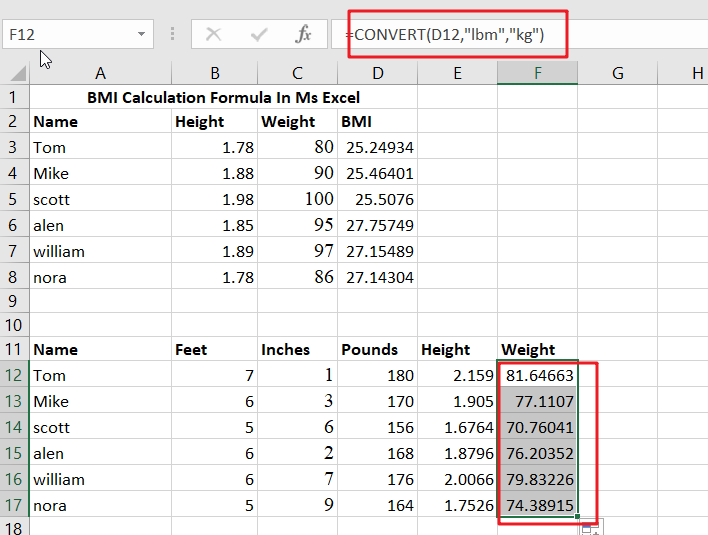Finally, we may use the usual BMI formula. In G12, the Formula is:

`=F12/E12^2/ compute BMI`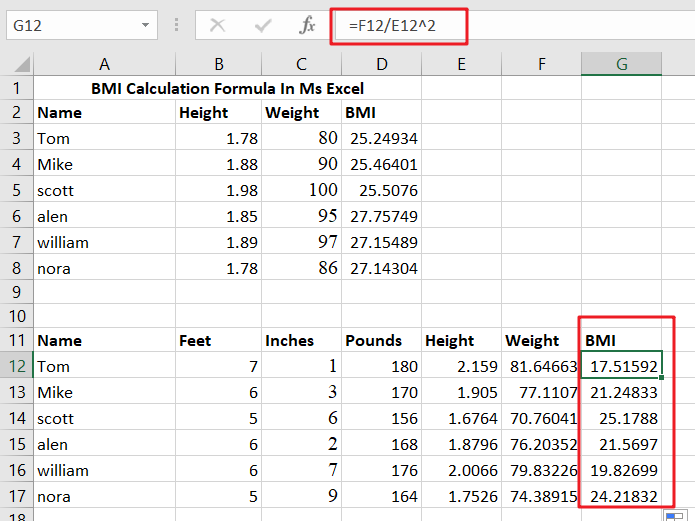We utilize Excel’s exponentiation operator, the caret () to square height.

In addition to the caret (), the POWER function may be used to raise to a power such as this:

`=F12/POWER(E12,2)`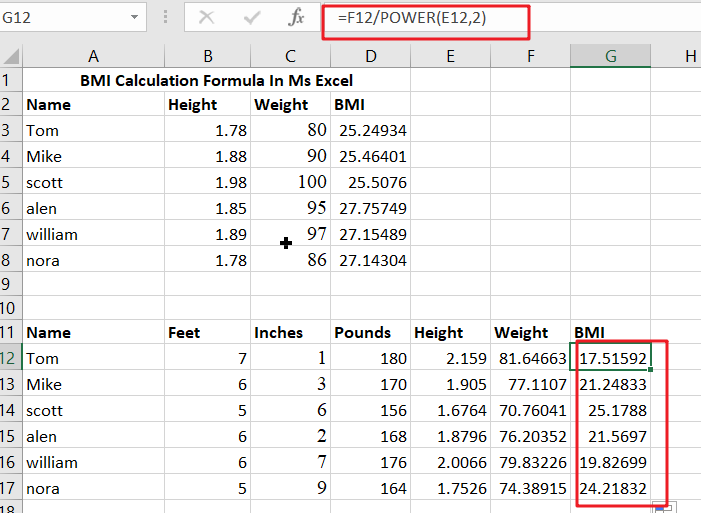## Alternatives

The formulae mentioned above can be simplified somewhat. We may use a single CONVERT function to determine the height, as seen below:

`=CONVERT(B12*12+C12,"in","m")`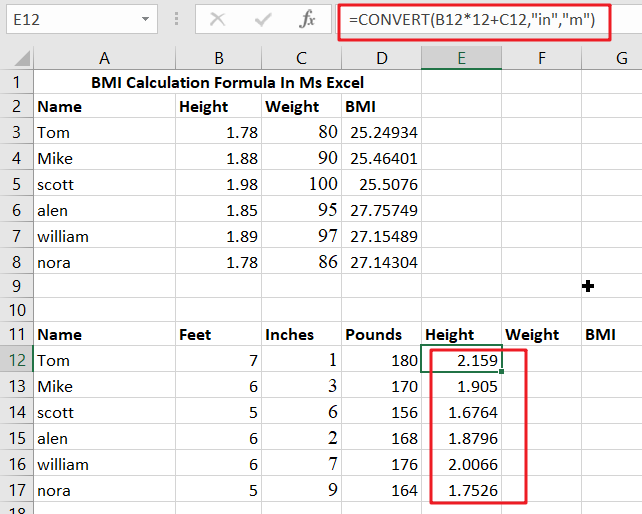To put it another way, we convert feet to inches immediately within the function parameter. This process occurs before the CONVERT function is called when Excel evaluates the calculation. It’s not as readable, but it’s more compact.

Similarly, we might use CONVERT to convert inches to feet as follows:

`=CONVERT(B12+C12/12,"ft","m")`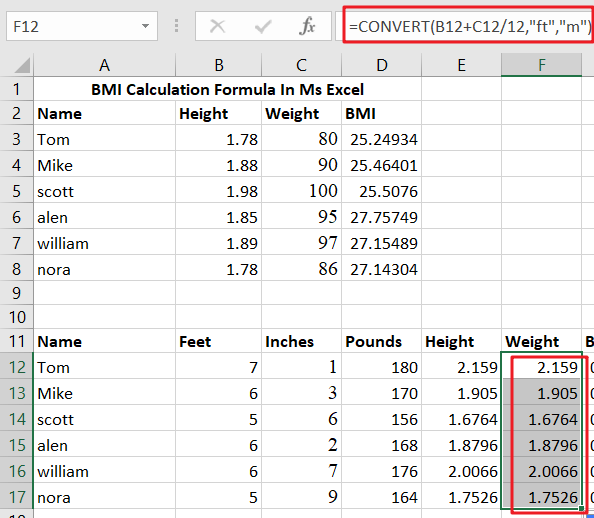The end outcome is the same as before. The critical feature is that you may nest additional calculations directly in a function parameter.

Sidebar